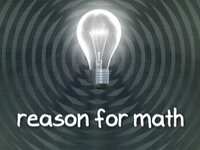# Reason for Math Volume 1: The Language of Math

Reason for Math is not your ordinary movie about Mathematics. Yes, it teaches you about mathematics but the main goal is to facilitate the understanding and general concepts of this science. Maths is a way of thinking and has a language of its own. So learning the vocabulary of this foreign language is key to understanding mathematics.

Volume 1 of „Reason for Math“ deals with the basic patterns in addition, subtraction, multiplication and division including:

• order of operations
• factors and multiples
• prime numbers and factoring into primes
• use of punctuation in math statements
• the distributive property

However, the real content of the video is how these basic ideas come together to help form the overall language of mathematics. We’ve all heard math called “the universal language”. This video examines how math is similar to word languages, yet has a unique structure all its own. We’ll see how the seemingly strange terminology (like “commutative” and “associative”) and apparently pointless factoids (like “the number 1 isn’t a prime number” ) aren’t simply data to memorize for a test, but are actually the foundation for logic and reason for the amazingly empowering language of math.

Some insights:

The content of Reason for Math, Volume 1 generally fits into national standards for 3rd through 5th grade level math. However, these standards bring the assumption that at a certain age, a student should understand a certain concept. Many learners can progress through their entire math education and have little understanding of the nature of the language of math and what it means to think mathematically.

This video is intended to introduce ideas to young students as well as eliminate confusion and misconceptions in older math-skeptical or math-fearful students.

Different interpretations of “There is a huge banana sale”:

### The 15 chapters of “Reason for Math”

• Abstract
• The Music of Math
• The Language of Math
• Encoding
• Order
• Rewording Relationships
• Writing Multiplication and Division
• Multiplication and Division Principles
• Factors and Multiples
• Factoring into Primes
• Punctuation
• The Distributive Property
• Patterns
• Problems?

Associating mathematical principles with easily remeberable images helps student to feel a deep understanding of what mathematics is. Learing the mathematical vocabulary is as important as understanding the construction of mathematical “sentences”. This video provides the appropriate connections for students.Director
Beau Janzen
Genre
Animated Movies
Mathematical Topics
Logic
Set theory
Number theory
Tags
Arithmetic
Numbers
For Children
Duration
97 minutes
Year of Release
2012
Languages
English
Similar Contents
Film: Flatland
Film: Hard Problems
Film: Flatland 2: Sphereland
Film: The Penrose Tessellation
Film: Fermat's Last Tango